# Plot ranges of data in R

21 Feb 2013
2013/02/21

How to control the limits of data values in R plots.

R has multiple graphics engines.  Here we will talk about the base graphics and the `ggplot2` package.

We’ll create a bit of data to use in the examples:

`one2ten <- 1:10`

`ggplot2` demands that you have a data frame:

`ggdat <- data.frame(first=one2ten, second=one2ten)`

Seriously exciting data, yes?

## Default behavior

The default is — not surprisingly — to create limits so that the data comfortably fit.

### Base

Figure 1 shows the default limits.  The command to do this is (almost):

`plot(one2ten, one2ten)`

The actual commands to create this figure and others are below in Appendix R.

Figure 1: Default base graphics plot.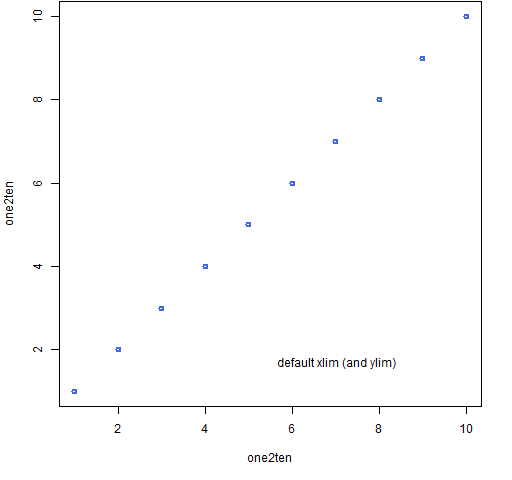### ggplot2

The default here is basically the same, though the resulting picture looks rather different.  Figure 2 is created with the commands:

```require(ggplot2)
print(qplot(first, second, data=ggdat))```

The `require` command makes sure that the package is loaded into the current R session.  In this setting graphs are objects, and they are rendered only when they are printed — hence the call to `print`.

Figure 2: Default `ggplot2` plot.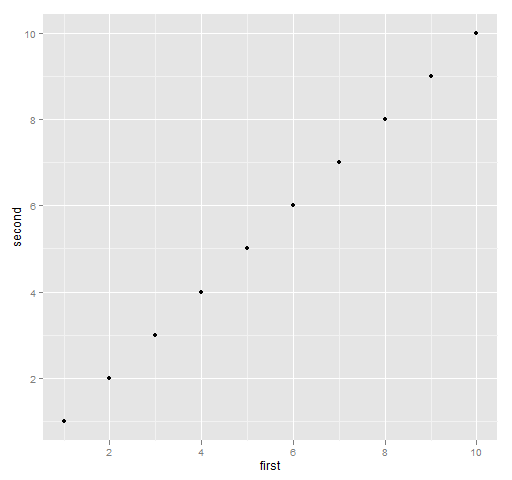## Typical limit

If the default isn’t what you want, you can change it.  Here we want — for some reason — more room on the left of the plot.

### Base

The simplified version of the command for Figure 3 is:

`plot(one2ten, one2ten, xlim=c(-2,10))`

Figure 3: Typical use of the `xlim` graphics parameter.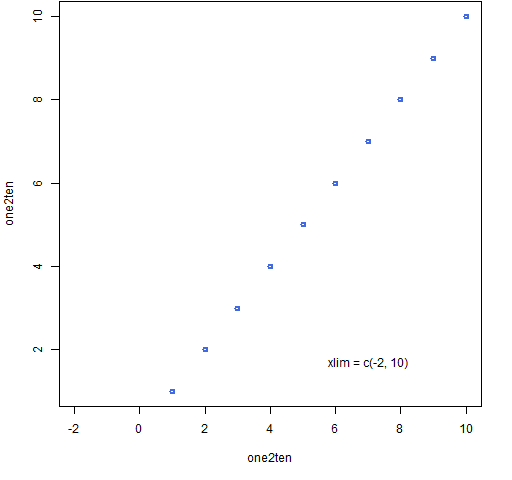The examples here are on the x-axis.  To control the y-axis, just substitute “y” for “x” — `ylim` rather than `xlim`.

### ggplot2

In `ggplot2` modifications or additions to a plot object are usually done by adding new terms:

`print(qplot(first, second, data=ggdat) + xlim(-2, 10))`

Figure 4: Typical `ggplot2` specification of x limits.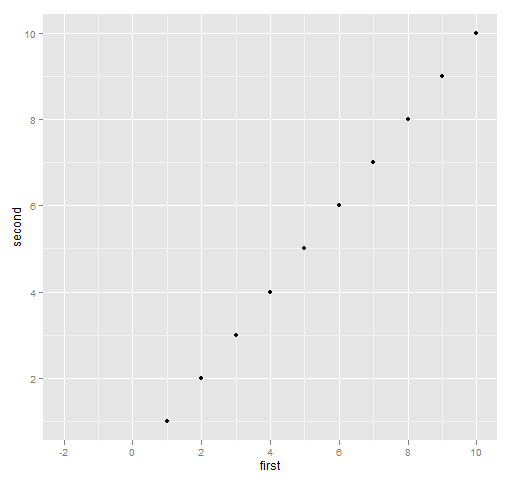## Reverse direction

The first limit need not be the smallest — if not, then the axis is reversed.

### Base

Figure 5: Base graph with the x-axis reversed.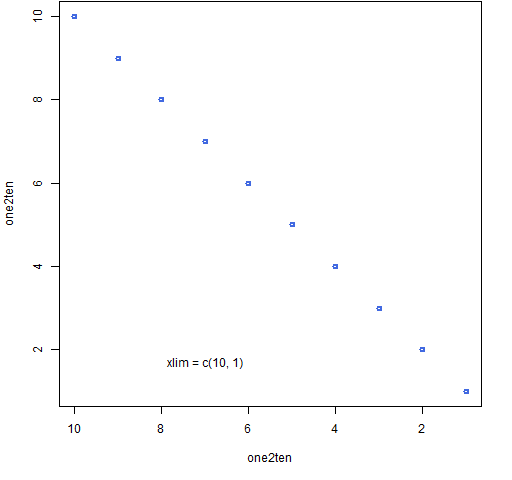### ggplot2

The command for Figure 6 is:

`print(qplot(first, second, data=ggdat) + xlim(10, 1))`

Figure 6: `ggplot2` with the x-axis reversed.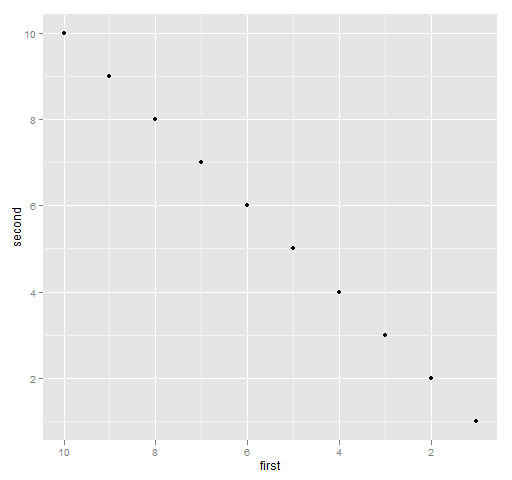## Expansion

The sharp-eyed will have noticed that the actual limits in the plots above are not what is specified.  The specifications are strictly inside the plots.  This makes it easy to make sure that no data is plotted on the boundary of the plot.

It is possible to change this behavior as well.

### Base

We can see what the real range is by looking at the `usr` graphics parameter:

```> plot(one2ten, one2ten, xlim=c(0,10))
> par("usr")
 -0.40 10.40  0.64 10.36```

You can set some graphics parameters, and some are read-only.  The `usr` parameter can be set, but you almost always want to just use it as is.  The first two elements of `usr` are the x-axis limits, the last two are the y-axis limits.

The x-axis was asked to have limits 10 apart, and we can see that there is an extra 0.4 on each side.

You can force the limits to be taken literally by specifying `xaxs` (or `yaxs` for the y-axis):

```> plot(one2ten, one2ten, xlim=c(0,10), xaxs="i")
> par("usr")
  0.00 10.00  0.64 10.36
> plot(one2ten, one2ten, xlim=c(0,10), xaxs="i", yaxs="i")
> par("usr")
  0 10  1 10```

The `"i"` stands for “internal”, the other (default) choice is `"r"` as in “regular”.

### ggplot2

Figure 7 is produced with:

```print(qplot(first, second, data=ggdat) +
scale_x_continuous(expand=c(0,0)))```

Figure 7: `ggplot2` with no x-axis expansion.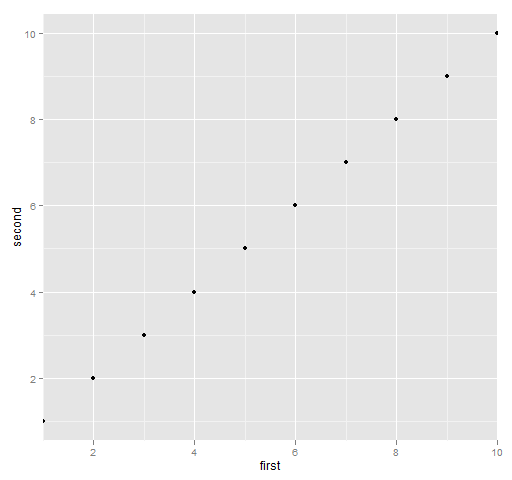I wasn’t able to both modify the expansion and `xlim` — I’m not sure if that is a bug or solely due to my ignorance of `ggplot2`.

## Appendix R

The actual commands that created the base graphics are:

Figure 1:

```plot(one2ten, one2ten, col="royalblue", lwd=2)
text(7, 1.7, " default xlim (and ylim)")```

Figure 3:

```plot(one2ten, one2ten, col="royalblue", lwd=2, xlim=c(-2,10))
text(7, 1.7, "xlim = c(-2, 10)")```

Figure 5:

```plot(one2ten, one2ten, col="royalblue", lwd=2, xlim=c(10,1))
text(7, 1.7, "xlim = c(10, 1)")```
Tags:
3 replies
1.Patrick Burns says:

Hadley relieved me of some of my ignorance with:
QUOTE

xlim(x) is just a shortcut for scale_x_continuous(limits = x)

so if you want to set both limits and expansion you need

scale_x_continuous(expand=c(0,0), limits = x)
END QUOTE

The following command does as I expect:

print(qplot(first, second, data=ggdat) +
scale_x_continuous(expand=c(0,0), limits=c(-2,10)))

2.Brandi Missouri Griffin says:

What if you have 2 vectors and you want your plot to automatically scale to the larger instead of the first vector?